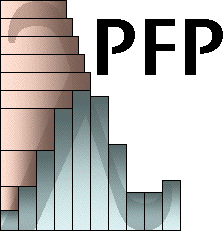A Probabilistic Functional Programming Library for Haskell Version: June 2006

The PFP library is a collection of modules for Haskell that facilitates probabilistic functional programming, that is, programming with stochastic values. The probabilistic functional programming approach is based on a data type for representing distributions. A distribution represent the outcome of a probabilistic event as a collection of all possible values, tagged with their likelihood.

Distributions can represent events, such as the roll of a die or the flip of a coin. For example, or example, the outcome of a die roll can be expressed as follows.

```die :: Dist Int
die = uniform [1..6]
```
The evaluation of die yields a distribution:
```> die
1  16.7%
2  16.7%
3  16.7%
4  16.7%
5  16.7%
6  16.7%
```
The function uniform takes a list of elements and produces a distribution where each element is equally likely. We can also use functions like uniform to construct probabilistic functions, called transitions. Here is a transition which, given a number, either adds one or doesn't with equal probability.
```succOrId x = uniform [x, x+1]
```
We could also represent this function using the choose operator which constructs a distribution of two elements.
```succOrId x = choose 0.5 x (x+1)
```
Imagine we want to roll a dice, then either add one or not. We can use monadic operators to combine probabilistic operations. This can be represented concisely, or in a more verbose way.
```droll = die >>= succOrId
```
Or:
```droll = do d <- die
succOrId d
```
The PFP library provides a set of function definitions that allow the declarative description and evaluation of probabilistic situations. These function can be employed in many different ways - be it solving of statistics problem from textbooks or the application to scientific problems. The approach is constructive by defining and applying probabilistic functions.

To use the library:

• Gunzip and untar the archive. This will create a directory pfp-jun06. Change to that directory.
```ghci Dice.hs
```
• Formulate queries. For example, what is the distribution of points when rolling two dice?
```*Dice> mapD (uncurry (+)) (prod die die)
7  16.7%
6  13.9%
8  13.9%
5  11.1%
9  11.1%
4   8.3%
10   8.3%
3   5.6%
11   5.6%
2   2.8%
12   2.8%
```
Or, what is the probability of the two dice differing by one point?
```*Dice> (==1) ?? mapD (abs . uncurry (-)) (prod die die)
27.8%
```
More examples are included in the papers listed below.

## Further Information

Probabilistic Functional Programming in Haskell, Martin Erwig and Steve Kollmansberger
Journal of Functional Programming, Vol. 16, No. 1, 21-34, 2006

Modeling Genome Evolution with a DSEL for Probabilistic Programming, Martin Erwig and Steve Kollmansberger
8th Int. Symp. on Practical Aspects of Declarative Languages, LNCS 3819, 134-149, 2006

Modeling Biological Systems wit FuSE, Martin Erwig and Steve Kollmansberger
A short tutorial, November 2005, under construction

A Domain-Specific Embedded Language for Probabilistic Programming, Steve Kollmansberger
Master's Thesis, December 2005

Computing probabilities is one thing, understanding how and why questions of a probablisitic nature give rise to their resulting probabilities is another. Consider, for example, the boys/girls riddle "Given that a family with two children has a boy, what is the probability that the other child is a girl?" It is not difficult to express this problem in PFP and compute the answer (see the file Boys.hs in the distribution). However, the result, namely 2/3, is unintuitive to many, and an explanation why this answer is correct is not part of the computation. The idea of Explanation-Oriented Programming addresses this problem by shifting the focus to the design of languages that not just produce results but explanations of how these results are obtained. The explanation of probablistic reasoning is specifically addressed in the following two papers.

Visual Explanations of Probabilistic Reasoning, Martin Erwig and Eric Walkingshaw
IEEE Int. Symp. on Visual Languages and Human-Centric Computing, 23-27, 2009

A DSL for Explaining Probabilistic Reasoning, Martin Erwig and Eric Walkingshaw
IFIP Working Conference on Domain Specific Languages, LNCS 5658, 335-359, 2009
Best Paper Award